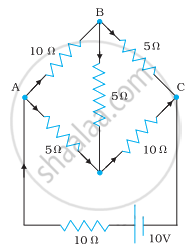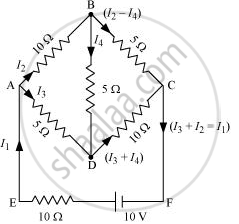# Determine the current in each branch of the network shown in fig. - Physics

Numerical

Determine the current in each branch of the network shown in fig.#### Solution

Current flowing through various branches of the circuit is represented in the given figure.I1 = Current flowing through the outer circuit

I2 = Current flowing through branch AB

I3 = Current flowing through branch AD

I2 − I4 = Current flowing through branch BC

I3 + I4 = Current flowing through branch CD

I4 = Current flowing through branch BD

For the closed-circuit ABDA, the potential is zero i.e.,

10 I2 + 5 I4 − 5 I3 = 0

2 I2 + I4 − I3 = 0

I3 = 2 I2 + I4 ….......(1)

For the closed-circuit BCDB, the potential is zero i.e.,

5(I2 − I4) − 10(I3 + I4) − 5I= 0

5 I2 + 5 I4 − 10 I3 − 10 I− 5 I= 0

5 I2 − 10 I3 − 20 I= 0

I2 = 2 I3 + 4 I…......(2)

For the closed circuit ABCFEA, potential is zero i.e.,

−10 + 10 (I1) + 10 (I2) + 5(I2 − I4) = 0

10 = 15 I2 + 10 I− 5 I4

3 I2 + 2 I− I4 = 2 ….........(3)

From equations (1) and (2), we obtain

I3 = 2(2 I+ 4 I4) + I4

I3 = 4 I+ 8 I4 + I4

−3 I3 = 9 I4

−3 I4 = + I…..........(4)

Putting equation (4) in equation (1), we obtain

I3 = 2 I+ I4

− 4 I4 = 2 I2

I2 = −2 I4 …..........(5)

It is evident from the given figure that,

I1 = I+ I2 ….........(6)

Putting equation (6) in equation (1), we obtain

3 I2 + 2(I+ I2) − I4 = 2

5 I2 + 2 I− I4 = 2 …........(7)

Putting equations (4) and (5) in equation (7), we obtain

5(−2 I4) + 2(−3 I4) − I4 = 2

− 10 I4 − 6 I− I4 = 2

17 I4 = −2

I4 = (-2)/17"A"

Equation (4) reduces to

I3 = −3(I4)

= -3((-2)/17)

= 6/17"A"

I2 = −2(I4)

= -2((-2)/17)

= 4/17"A"

"I"_2 - "I"_4 = 4/17 - ((-2)/17)

= 6/17"A"

"I"_3 + "I"_4 = 6/17 + ((-2)/17)

= 4/17"A"

I1 = I3 + I2

= 6/17 + 4/17

= 10/17"A"

Therefore, current in branch AB = 4/17"A"

In branch BC = 6/17"A"

In branch CD = (-4)/17"A"

In branch AD = 6/17"A"

In branch BD = ((-2)/17)"A"

Total current = 4/17 + 6/17 + (-4)/17 + 6/17 + (-2)/17 = 10/17"A"

Concept: Kirchhoff’s Rules
Is there an error in this question or solution?

#### APPEARS IN

NCERT Physics Part 1 and 2 Class 12
Chapter 3 Current Electricity
Exercise | Q 3.9 | Page 128
NCERT Class 12 Physics Textbook
Chapter 3 Current Electricity
Exercise | Q 9 | Page 128

Share